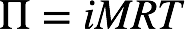# Problem: The osmotic pressure of a solution containing 7.0 g of insulin per liter is 23 torr at 25 °C. What is the molar mass of insulin?

###### FREE Expert Solution

Recall: The osmotic pressure Π of a solution is given by:where i = van't Hoff factor, M = molarity, R = gas constant, T = temperature.

Insulin is non-electrolyte solute, which means i = 1. The temperature given is 25 ˚C + 273.15 = 298.15 K. The osmotic pressure of the solution is 23 torr. Converting this to atm:###### Problem Details

The osmotic pressure of a solution containing 7.0 g of insulin per liter is 23 torr at 25 °C. What is the molar mass of insulin?